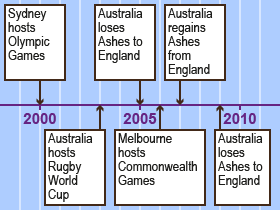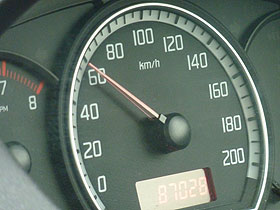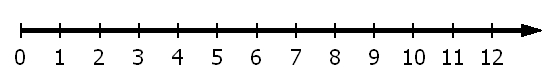Home > Patterns > Misunderstandings > Number lines

# Number lines

There are many instances when numbers are represented on a line. Common examples are rulers and other measurement scales, timelines, analogue clocks and the axes of graphs. They are all examples of number lines. Below are two examples of number lines. Notice that a number line can be curved.Some recent Australian sporting events.A speedometer.Source: Sean MacEntee. Licensed under a Creative-Commons Attribution 2.0 Generic (CC BY 2.0).

Number lines all have one simple pattern: the numbers are equally spaced.

Some numbers are marked, and these are equally spaced.

Also, some of the marks are labelled with numbers, and these marks are again equally spaced.

In a simple number line, every number is shown and labelled.A number line.

The arrow indicates that the number line can be continued indefinitely.

A number line is a repeating pattern; the unit of repeat is the segment between two marks.

Students can learn about number lines at the same time that they are learning about repeating patterns.

For example, the marks and labels on a number line can be obtained by skip counting — common intervals are two, five or ten.

Reading off intermediate points on a number line requires the user to divide a space into an equal number of parts.

This process can be linked to linear models of fractions.

## Drawing and reading number lines

Here are some examples of the difficulties students experience if they do not understand the structure of the number line or number scale.

## Number lines and repeating patterns

Students will understand the number line better if they practise representing skip counting and plotting timelines. Both activities emphasise that the numbers on a number line are equally spaced.

## Drawing an analogue clock face

By drawing analogue clock faces, students can learn a great deal about number lines as well as time measurement.